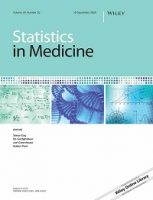# Layman’s abstract for Statistics in Medicine article on A puzzle of proportions: Two popular Bayesian tests can yield dramatically different conclusionsEach week, we publish layman’s abstracts of new articles from our prestigious portfolio of journals in statistics. The aim is to highlight the latest research to a broader audience in an accessible format.

The article featured today is from Statistics in Medicine with the full article available free to read here.

A puzzle of proportions: Two popular Bayesian tests can yield dramatically different conclusionsStatistics in Medicine20211– 15. doi:10.1002/sim.9278

If you are reading this, you are probably the type of person who likes puzzles. Here is one: In scenario A you observe two groups, one control and one treatment group, which both show y = 500 successful recoveries out of n = 1000 people each. In scenario B, you again observe one control and one treatment group with the same sample size n = 1000, but with both groups showing only y = 10 successful recoveries. Which of these scenarios provides stronger evidence for the null hypothesis that the two proportions are equal?

One way to quantify the evidence for the null hypothesis is by means of the Bayes factor, which pits the predictive performance of two hypotheses against each other. Bayesian hypothesis testing by means of the Bayes factor requires the specification of prior distributions for parameters. As it turns out, the Bayesian test that is widely used as the gold standard yields the opposite conclusion than a Bayesian test that we think is more suitable for such testing problems.

The most popular analysis approach views the comparison of proportions from a contingency table perspective, assigning prior distributions directly to the two proportions. Another, less popular approach views the problem from a logistic regression perspective, assigning prior distributions to logit-transformed parameters.

The most widely used test yields a Bayes factor of about 18 for scenario A and 88 for scenario B. The logistic test yields a Bayes factor of about 11 for scenario A and 3 for scenario B. A reversal!

To show the impact of this in practice, the work reanalyzes 39 null results from the New England Journal of Medicine with both approaches, indeed finding that the two tests can lead to markedly different conclusions, especially when the observed proportions are at the extremes (i.e., very low or very high). The article explains these stark differences and provides recommendations for researchers interested in testing the equality of two proportions and users of Bayes factors more generally. The test that assigns prior distributions to logit-transformed parameters creates prior dependence between the two proportions and yields weaker evidence when the observations are at the extremes. When comparing two proportions, the article argues that this test should become the new default.